# Easy sentence similarity with BERT Sentence Embeddings using John Snow Labs NLU

1 Python line to Bert Sentence Embeddings and 5 more for Sentence similarity using Bert, Electra, and Universal Sentence Encoder Embeddings for Sentences

This tutorial shows you how easy it is to get the latest Bert Sentence Embeddings using John Snow Labs NLU in just 1 line of code.
With these Embeddings, we will compare every sentence pair in a Stack Overflow Question dataset and find the most similar ones. We will also see how simple it is, to find the most similar sentence in our dataset, to a new given sentence.
In addition, we will also show how to leverage 3 Sentence Embeddings at The same time, BERT, Universal Sentence Encoder, and Electra Embeddings to tune our similarity results, it does not take more than 10 lines, promise!

With all these tools, it is surprisingly simple to build question answering systems and similar applications

More precisely, we will see how you can implement the following things with NLU :

1. How to get sentence Bert, USE, Electra sentence embeddings using BERT
2. How to find N most similar sentences in a dataset for a given sentence in the dataset using BERT
3. How to calculate the similarity matrix and visualize it for a dataset using BERT
4. How to find the N most similar sentences in a dataset for a new sentence using BERT
5. How to find the N most similar sentences in a dataset for a new sentence using BERT, USE, Electra at the same time!

# 0 . What is Sentence similarity and why is it useful?

Sentences are made up of words and raw words are difficult to compare to each other. Various research has been made to create meaningful numerical vector representations of words. With the most recent breakthroughs in deep learning, especially in the Transformer area with models like BERT incredible tools have developed to solve the problem of creating these vectors and they encode incredible amounts of meaning and context in them.
This can be leveraged to build various kinds of NLP applications, like question-answering systems, and can be applied to many domains.

# 0.1 Install NLU

To run NLU you need Java 8 and Spark NLP installed. The following script will setup everything you need to get started with Spark NLP on Google Collab or Kaggle. Follow the Docs for further info

`import os ! apt-get update -qq > /dev/null    # Install java ! apt-get install -y openjdk-8-jdk-headless -qq > /dev/null os.environ["JAVA_HOME"] = "/usr/lib/jvm/java-8-openjdk-amd64" os.environ["PATH"] = os.environ["JAVA_HOME"] + "/bin:" + os.environ["PATH"] ! pip install nlu import nlu`

# 0.2 Get some data

We will download a dataset from the “60k Stack Overflow Questions with Quality Rating” Kaggle dataset. This simple wget command downloads the dataset to our /tmp dir and in the next line, we read it to a pandas df.

`import pandas as pd# Download the dataset! wget -N https://ckl-it.de/wp-content/uploads/2020/11/60kstackoverflow.csv -P /tmp# Load dataset to Pandasdf = pd.read_csv('/tmp/60kstackoverflow.csv')df`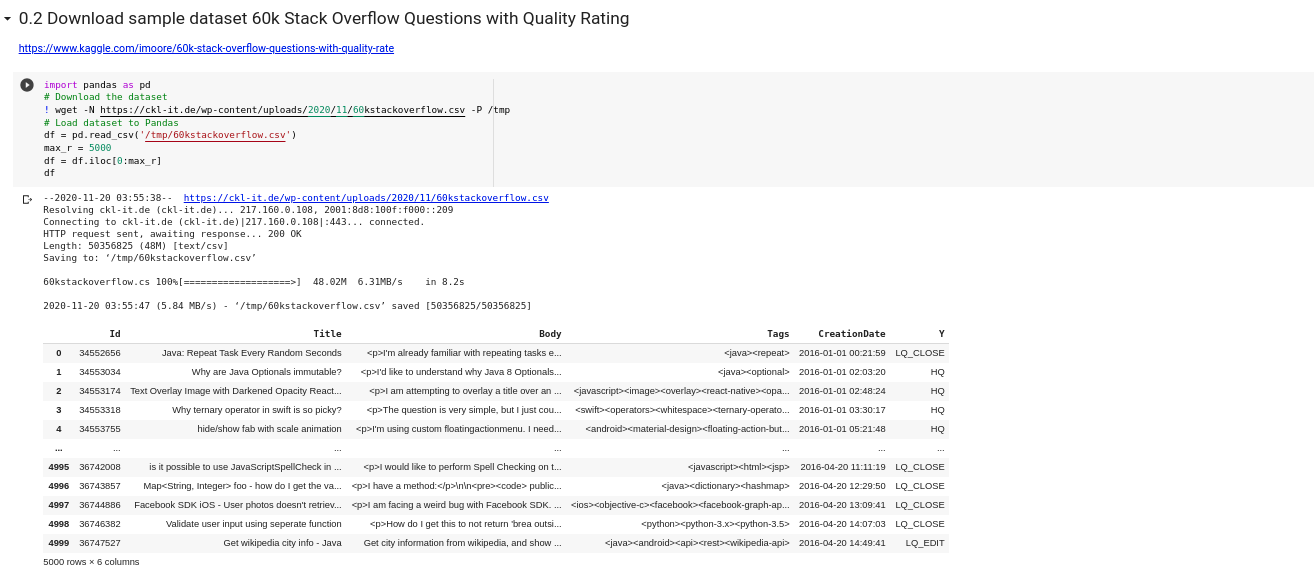We will use the first 5k questions in the dataset to save time and RAM

# 1.1 Generate Bert Sentence Embeddings with NLU

First, we load the Bert Sentence Embeddings pipeline via nlu.load() and then pass the column which contains the question Titles we want to embed to the pipe.predict() function.

`import nlupipe = nlu.load('embed_sentence.bert')predictions = pipe.predict(df.Title,] output_level='document')predictions`

# 2.1 Get the most similar sentences for a sentence in our dataset

The following code calculates the similarity between every sentence pair in the dataset and stores it in the sim_mat variable. sim_mat[i][j] represents the similarity of sentence in the df.iloc[i].Title to the sentence df.iloc[j].Title.
Thus, sim_mat[i] is a vector of the similarities of a sentence i to every other sentence j in the dataset, i.e. df.iloc[i].Title to every df.iloc[:].Title.

`from sklearn.metrics.pairwise import cosine_similarityimport numpy as np# put all sentence embeddings in a matrixe_col = 'embed_sentence_bert_embeddings'embed_mat = np.array([x for x in predictions[e_col]])# calculate distance between every embedding pairsim_mat = cosine_similarity(embed_mat,embed_mat)#get sim score for a given sentence at position df.iloc[sentence_id]sentence_id = 0print("Similarities for Sentence : " + df.iloc[sentence_id].Title)# write sim scores to dfdf['sim_score'] = sim_mat[sentence_id]sim_df.sort_values('sim_score', ascending = False)`

The code can be summarised in a function and which generates a dataframe for the sentence at position df.iloc[sentence_id] to every other sentence in the Dataframe and returns a column named ‘sim_score” based on that. We can sort that dataframe on the sim_score column descending, to see the most similar sentences in our dataset, for the sentence at df.iloc[sentence_id].

# 2.2 Define helper function for plotting similarity between one sentence and every other sentence in the dataset

The following function lets us play around and just plug in a few different sentence positions we want to calculate the similarities for. The the 0'th sentence we find lots of Java results!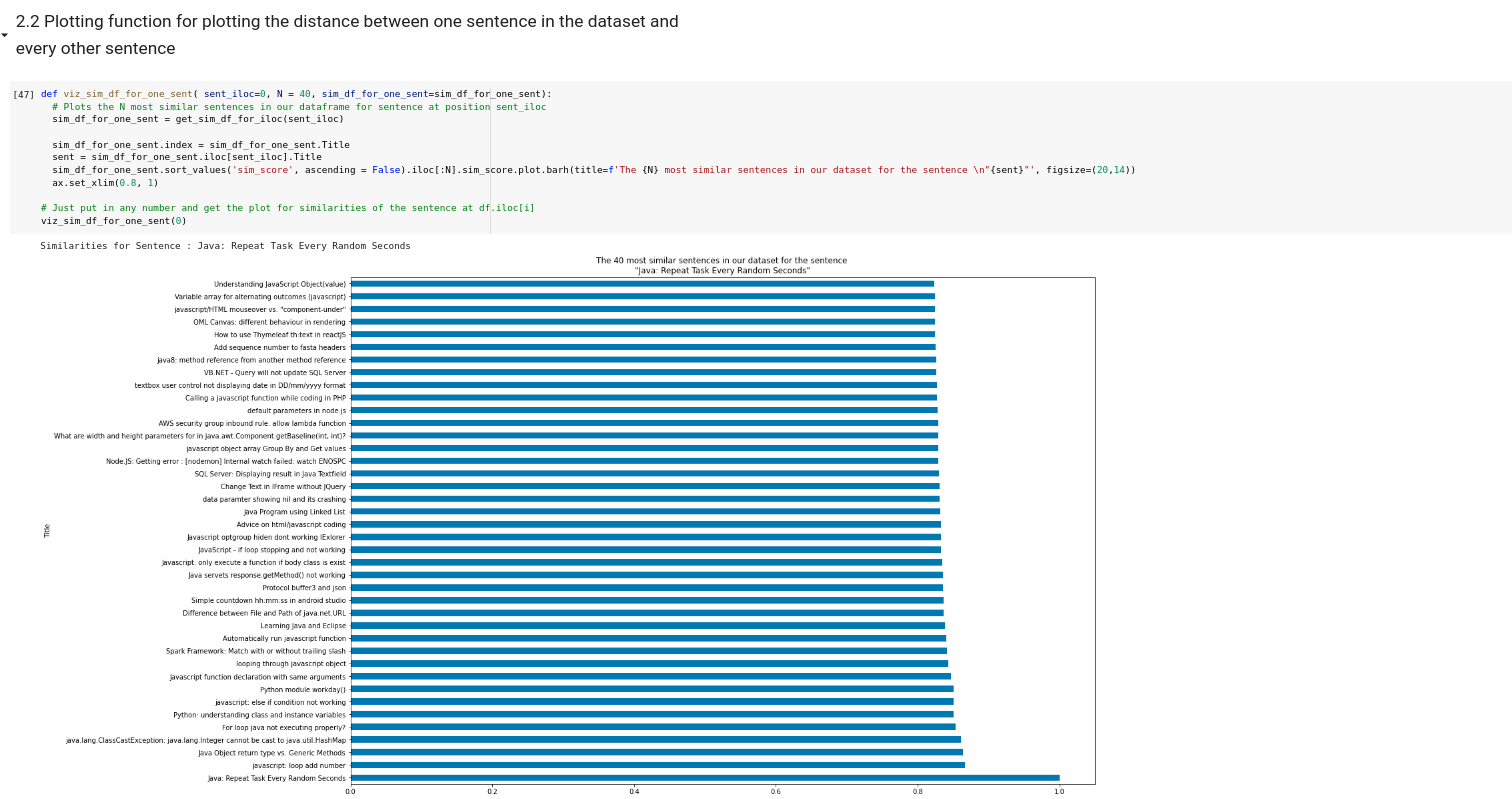The 40 most similar questions for the question “Java: Repeat Task Every Random Seconds”. Lots of Java!

# 3.1. Calculate pairwise distances between Sentence Embeddings and generate a similarity matrix

If you want to encode the similarity of every sentence to every other sentence as a column in your data frame, the following code snippet is helpful. It will create a new column for every sentence in the dataset and write the similarity of that particular sentence to its corresponding column.

This function calculates the distances of every sentence pair, creates forever sentence a new column, i_sim the represents the similarity of sentences at predictions.iloc[i] to every other sentence j

`from sklearn.metrics.pairwise import cosine_similarityimport numpy as np## Calculate dinstance between all pairs of sentences in DF# drop any NApredictions.dropna(inplace=True)# put embeddings in matrixe_col = 'en_embed_sentence_small_bert_L12_128_embeddings'embed_mat = np.array([x for x in predictions[e_col])# calculate distance between every embedding pairsim_mat = cosine_similarity(embed_mat,embed_mat)df['sim_score'] = sim_mat[sentence_id]`

# 3.2 Define Helper function to plot similarity matrix for the first N sentences in the dataset

The following method takes in a dataframe that has only columns with similarity scores, that is why we remove them first. It plots the heatmap for the similarities of the first N sentences in the dataset

# 3.3 Plot similarity matrix for all sentences starting at some index until some end index

The following method plots the similarity matrix for the sentences at start and end iloc positions.

# 4.1 Find the N most similar sentences in a datset for a new sentence that does not exist in the data using BERT

To find the most similar sentences in our dataset for a new string we can use this code snippet. For the question “How to get started with Machine Learning in Python” we actually get some matching results. Keep in mind, we just used a subset of the dataset to compute this, since it takes quite a while.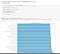The N most similar sentences for the question “How to get started with Machine learning in Python”. The results re not great but we will improve them in the next steps

# 4.2 Define Helper plotting function to plot results of embedding a string

This method embeds and visualized the similarities for one string right away for us, enabling maximum fun in 1 line :)

# 5.1 Multi Embedding Similarity, find the N most similar sentences in a dataset for a new sentence using BERT, USE, Electra

We can use NLU to load multiple different embeddings at the same time and accumulate their distances for every sentence to derive a new distance metric which can potentially improve results, since each Transformer Embedding model has been trained differently and on different datasets.

This downloads us 3 of the latest sentence Embeddings at the same time, BERT, Universal Sentence Encoder, and Electra Embeddings

`multi_pipe = nlu.load('en.embed_sentence.electra embed_sentence.bert use')multi_embeddings = multi_pipe.predict(df.Title,output_level='document')`

# 5.2 Multi Embeddings Similarity calculation

Now that we have all 3 embeddings generated for every sentence, the following code snippet calculates for a given input string the distances to every sentence in the dataset, while summing up the distances of the 3 different embeddings and dividing by 3 to normalize between 0 and 1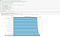We can already see better results for the question “How to get started with Machine learning in Python” when using more embeddings!

# 5.3 Define helper function to plot the similarity results of a multi embedded string

The following method let’s us input a string and find the most similar string in the dataset, very handy to play around and have some fun with and compare with the previous results!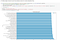Lots of array results for “How to sort an Array in Java”

# 6 There are many more Sentence Embeddings!

Various languages and even multilingual sentence embeddings are available in NLU!

# 8. References

Notebook for article